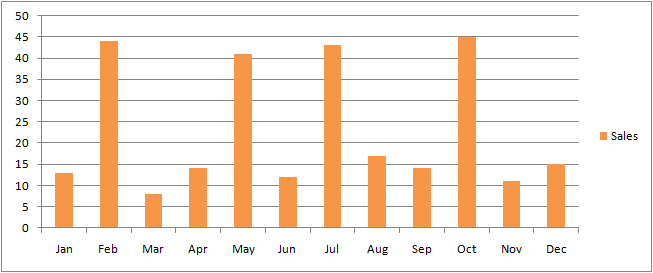We have published an introductory tutorial that provides the formula used to compute the reorder point based on the forecasted demand, the demand uncertainty, the lead time and a couple of other factors.

This classical safety stock calculation comes with a couple of key assumptions about the demand. We have already posted about how to handle varying lead time. Then, there is another implicit assumption in the classical formula: buyers are assumed to be independent.

Recently, we have been approached by a company that frequently sell items in bulk to classrooms. Although most of the sales are single-item order, from time to time, comes a 20-30 items order for a whole classroom. The graph below illustrate the resulting sales patterns of intermittent bulk purchase.

Dislaimer: numbers made up and some results are widly approximated for the sake of simplicity.Over those 12 months, we can see that we have 2 patterns:

• ongoing single-unit orders which account for 13 orders per months on average.
• intermittent bulk sales which account for +30 orders on average.

The average monthly sales is at 23 orders per month, but if we remove the bulk order factor, then the average purchase drop to 13 orders per month.

Now, what is the right safety stock in this situation? If we consider the classical safety stock formula with typical settings, then we are going to have a reorder point established at roughly 30 items: the 23 orders per month average, plus the safety stock itself covering the demand uncertainty. Bulk purchases at 30 items are very likely to be missed short of a hand-few items.

Yet, the classical safety stock calculation is less than optimal: here, we end-up storing about twice as much items than we need to to address the individual purchase, and yet, the safety stock is not high enough to cover big bulk purchases.

In order to address bulk purchases, we need to refine our safety stock formula to take this pattern into account. For sake of simplicity, we are going to model the bulk purchase pattern as a single factor later reintegrated in the safety stock formula.

In order to reflect the bulkiness of the sales, we could consider the largest purchase for each item being sold. Yet, this value is not robust in the statistical sense, as a single super-large historical purchase can completely skew the results.Instead, we should rather consider a quantile of the bulk order quantity distribution as illustrated by the threshold Q in the illustration here above where all orders have been ordered from the smallest quantity to the largest one.

In this safety stock analysis, there is a natural fit for the quantile value to adopted for Q: it should be equal to the service level - as defined for the classical safety stock formula - that is to say the desired probability of not having a shortage.

Let call `y``Q` the bulk quantity associated to probability Q (in this illustration here above, we have `y``Q` = 30). Technically, `y``Q` is the inverse cumulative distribution of sales function taken at the quantile Q. The reorder point calculation becomes:

```R = D + MAX(σL * cdf(P); y``Q``) ```

where `σL * cdf(P)` happens to be the safety stock as computed based on the demand uncertainty.

Computing `y``Q` in Excel is a bit tedious because there is not equivalent of the PERCENTILE function for inverse cumulative distribution. We have to resort either to an histogram scheme or to a VBA macro.

The ICMD User Defined Function for Excel, pasted below, performs the `y``Q` calculation, assuming the sales orders are listed in an Excel range and sorted in increasing order.

’ Inverse cumulative distribution
Function ICMD(r As Range, q As Double)
’ Computing the total
Dim s As Double
For Each c In r
s = s + c.Value
Next
’ Finding the threshold
Dim a As Double
For Each d In r
a = a + d.Value
If a >= (q * s) Then
ICMD = d.Value
Exit For
End If
Next
End Function

Based on this refined formula applied to the sample data, we obtain a reorder point R = 13 (demand forecast) + 30 (bulk quantity) = 43 which is sufficient to address the bulk purchase with a high probability while keep the inventory as low as possible.